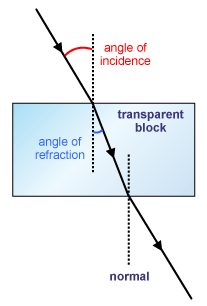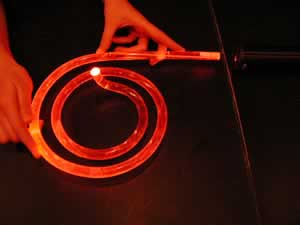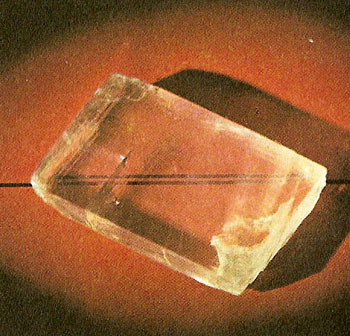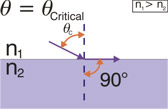A# refractionFig 1. Refraction of a light ray on entering and leaving a glass block.Fig 2. When light passes from one transparent substance to another, its speed changes. The bending which occurs when a beam of light crosses the boundary (between, for example, air and glass) obliquely, can be explained on the basis of the change in speed; one side of the beam is affected before the other. In the illustration, a beam passes through two glass blocks, first at right angles and then at an oblique angle.

Key
a) The length of a chosen number of wavelengths of the light in the air. Due to the reduction in speed, the corresponding length in glass is a/1.5, = 2/3a; the figure 1.5 is the refractive index of the glass.
i) The angle at which the light beam reaches the surface of the glass.
r) The angle at which the beam enters the glass.
b) The distance which the right-hand side of the beam travels in air after the left-hand side of it has entered the glass. The corresponding distance in the glass, as before, is b/1.5. From the two outlined right angled triangles, it can be seen that (sin i) / (sin r) = the refractive index 1.5; this is the basic law of refraction.Fig 3. Total internal reflection in an optical cable.Fig 4. Double refraction displayed by a crystal of Iceland spar.

Refraction is the change in direction of a ray of light from its original path as it passes from one transparent material to another with a different refractive index. This index is defined as equal to 1 in a vacuum. For any other medium it is defined as the speed of light in a vacuum divided by the speed of light in the medium. Thus refraction occurs when the speed of light is different in the two media. The amount by which rays of light change direction depends on the media involved and the frequency of the light.

The angle of refraction depends on the light's frequency. Different frequencies refract at slightly different angles – a phenomenon known as dispersion. The most familiar example is the dispersion of white light into a spectrum of colors when it passes through a prism.

The phenomenon of refraction is used in the design of lenses and prisms, and of combinations of lenses such as eyepieces and refracting telescopes. In nature, refraction causes objects to appear higher in the sky than they otherwise would. Objects more than halfway from the horizon to the zenith (i.e., with an altitude greater than 45°) are almost totally unaffected; however, objects near the horizon can be shifted by a degree or so. It is also responsible for the well-known phenomena of rainbows, mirages, and atmospheric haloes.

## Refractive index

The refractive index is the ratio of the speed of light in a vacuum to the speed of light through another medium. It is always greater than one, unless in a perfect vacuum, as the presence of matter retards the speed of light. When passing through a medium, long wavelengths refract less than short wavelengths, so the refractive index of a medium depends on the wavelength of light passed through it.

A refractometer is an instrument that measures the refractive index of liquids for a particular color of light; the index is often very sensitive to impurities besides allowing easy determination of the proportions present in two-component mixtures such as water and ethanol.A handheld refractometer.

## Critical angleThe critical angle is the smallest angle of incidence, at a refractive boundary, at which total internal reflection occurs. In telecommunications, it is the angle at which a radio wave just misses being reflected by the ionosphere.

## Total internal reflection

Total internal reflection is the reflection that occurs when light strikes an interface at an angle of incidence (with respect to the normal) greater than the critical angle (Fig 3). Total internal reflection occurs when, according to Snell's Law, the internal angle of incidence does not have a corresponding, real-valued, external angle of incidence. At angles less than the critical angle, both reflection and refraction occur.

## Double refraction

Double refraction, also called birefringence, is the splitting of a light wave into two waves, traveling at different speeds, by an optically anisotropic medium such as a crystal of Iceland spar (calcite), quartz, or topaz (Fig 4). In the phenomenon of double refraction a ray of unpolarized light is split into two rays plane-polarized (see polarization) at right-angles to each other. One is refracted in the normal way, the other with a refractive index that depends on the original direction of the ray.